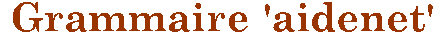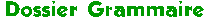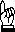Liste des mots composés français au singulier et au pluriel (175-7K)

A B C D E F G H I J K L M N O P Q R S T U V W X Y Z

( * = invariable)

kif-kif = kif-kif *
kilo-octet = kilo-octets
K-O = K-O * = knock-out *
kung-fu = kung-fu *
K-way = K-way *

A B C D E F G H I J K L M N O P Q R S T U V W X Y Z